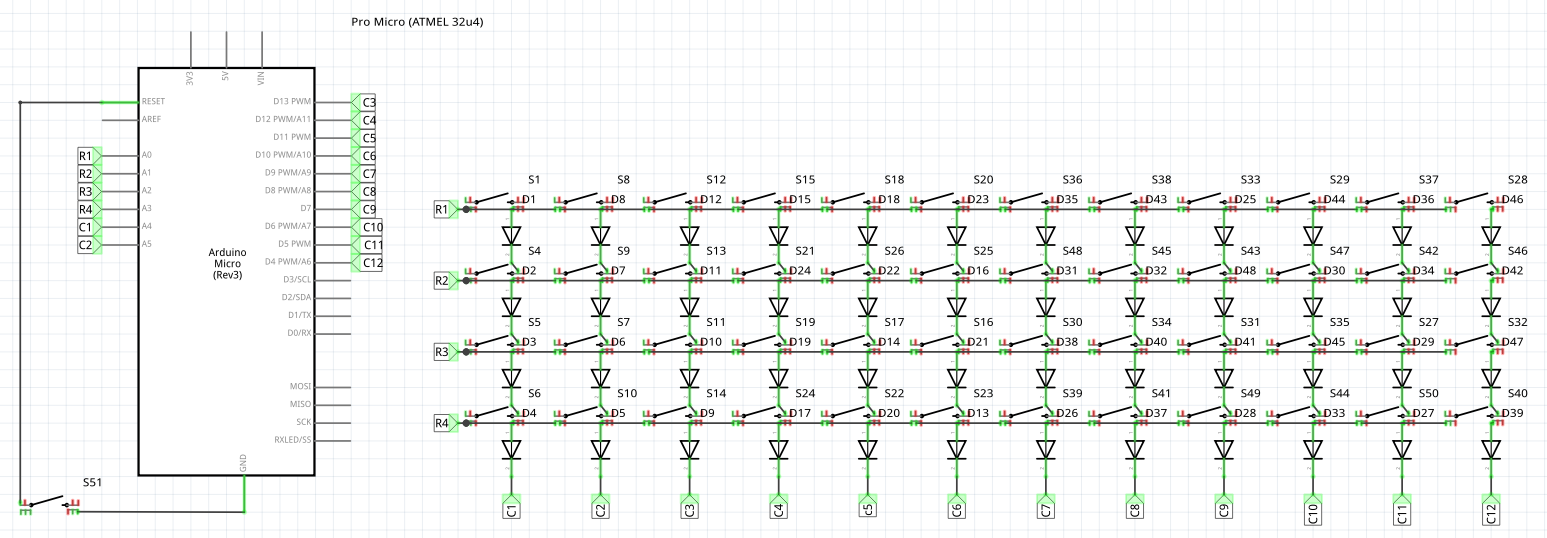# Matrix Keyboard

I'm trying to program a mechanical keyboard running on the Arduino Pro Micro (5V/16MHz). I'm using a matrix approach (4x12), and while I believe the code and the wiring (both of which I've checked multiple times) are correct, I can't get the first two rows to work no matter what. I've tried wiring it up to other pins, which works, but only for two rows at a time, when I hook up all the 4 rows, the two ones on top don't work. The code:

``````/*cols and rows*/
byte rows[] = {2,3,4,5};
const int row_Count = 4;
byte cols[] = {6,7,8,9,10,14,15,16,18,19,20,21};
const int col_Count = 12;

byte keyboard[col_Count][row_Count];

/*do the columns*/
for (int col_Index=0; col_Index < col_Count; col_Index++) {
/*setup for registering the column state*/
byte cur_Col = cols[col_Index];
pinMode(cur_Col, OUTPUT);
digitalWrite(cur_Col, LOW);

/*do the rows*/
for (int row_Index=0; row_Index < row_Count; row_Index++) {
byte row_Col = rows[row_Index];
pinMode(row_Col, INPUT_PULLUP);
pinMode(row_Col, INPUT);
}
/*disable the column*/
pinMode(cur_Col, INPUT);
}
}

void print_layout() {
for (int row_Index=0; row_Index < row_Count; row_Index++) {
Serial.print(row_Index);
Serial.print(F(": "));

for (int col_Index=0; col_Index < col_Count; col_Index++) {
Serial.print(keyboard[col_Index][row_Index]);
if (col_Index < col_Count) Serial.print(F(", "));
}
Serial.println("");
}
Serial.println("");
}

void setup() {
Serial.begin(115200);
delay(2000);
/*init the rows*/
for(int i=0; i<row_Count; i++) {
pinMode(rows[i], INPUT);
}
/*init the cols*/
for (int i=0; i<col_Count; i++) {
pinMode(cols[i], INPUT_PULLUP);
}
}

void loop() {
print_layout();

}
``````

This is the schematic, a "dirty sketch":• `two ones on top` is meaningless ... how are they connected? Jan 19, 2022 at 1:45
• have you checked if a matrix keypad library is available? Jan 19, 2022 at 2:48
• Did you "rotate" the rows, to see whether the port pins or the matrix give the issue? Did you try with a smaller number of rows, and increase it one by one? Why do you change the pin modes of the rows, they can always be `INPUT_PULLUP`? -- Unrelated: the condition in `print_layout()` does not prevent the printing of the last comma. `col_Index` is always lower than `col_Count` there. -- Unrelated: You can automate the calculation of the sizes, for example `const int col_Count = sizeof cols / sizeof cols;`. Jan 19, 2022 at 7:01
• @jsotola I made a dirty sketch, "two ones on top" refers to R1 and R2, and I'm not using the keypad library because I think it is unnecessary and apparently multiple key presses at the same time don't register. Jan 19, 2022 at 15:27
• @thebusybee I've tried switching around the row pins, there wasn't an issue with the "first two rows" in particular, the problem only began when I hooked up more than 2 rows to it, then only the "last two" would work. The idea with INPUT_PULLUP was to only check periodically whenever I wanted to, then switch to INPUT, I'm not sure if this makes sense because this is the first time I'm using this pinmode. Thanks for the other tips I've already implemented them. Jan 19, 2022 at 15:32